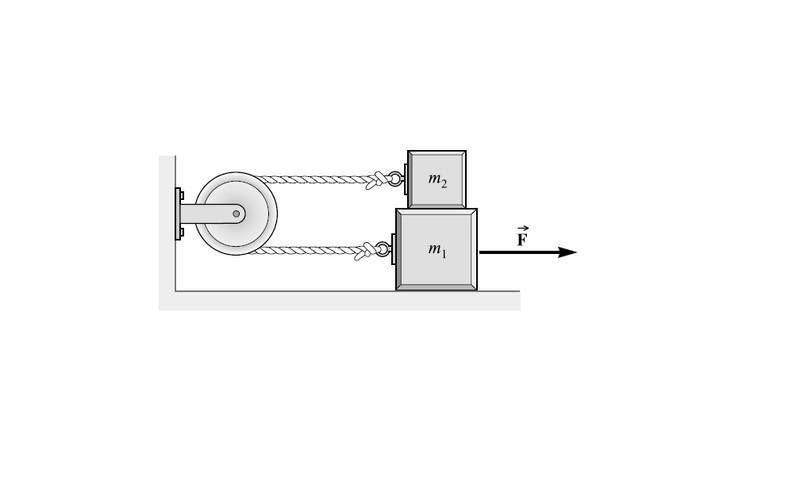# My solution doesnt match the Schaums Collgege Physics book.

udegatame

## Homework Statement

Imagine that a block of mass m2 is on top of block m1, which is on top a horizontal floor. m1>m2. A force F is applied to block 1 to the right. Both block1 and block2 are connected to the same pulley. In other words, one end of the pulley is connected to block1, and the other end of the pulley is connected to block2. The angle the cords make with each block is 0 degrees.For those of you with Schaum's College physics 10th edition, the question is chapter 3 excercise 35.

## The Attempt at a Solution

Let f be the coefficient of friction experienced by block2. In the free body diagram for block 2 (the block on top), I have developed the equation that Tension - f*m2*g =m2*a. But the book says that Tension = f*m2*g = m2*a. What have I done wrong?

udegatame
Here is a picture of the question.Mentor
In the free body diagram for block 2 (the block on top), I have developed the equation that Tension - f*m2*g =m2*a. But the book says that Tension = f*m2*g = m2*a. What have I done wrong?
I'd say you were correct.

Mentor
But the book says that Tension = f*m2*g = m2*a.
I think it's just a typo. That first = sign was meant to be a minus sign.

udegatame
Thanks Doc Al.